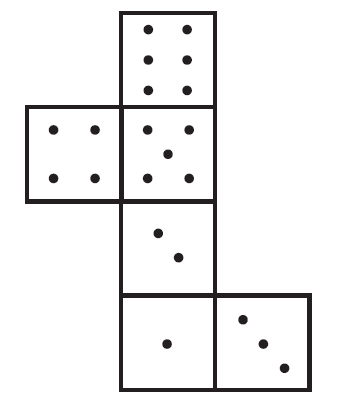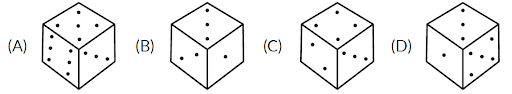Learn

# Cubes and Dice

17th Sep '2025 views6 mins read

 1 Introduction to Cube and Dice 2 Types of cubes 3 Facts about cube and net 4 Problems 5 Answers 6 Conclusion 7 References

18th September 2020

## Introduction to Cube and Dice

All you kids are familiar with the cube called dice which has been a part of our growing up years. Let’s explore the same dice a little more.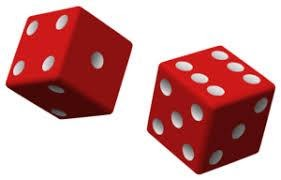• A Dice is a cube. Let us study some basic facts about Cube using the given figure.
• There are six faces in a cube and they are– \begin{align}ABCG, GCDE, DEFH, BCDH, AGEF\end{align} and \begin{align}ABHF\end{align}.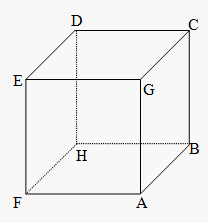• Four faces are adjacent to one face
• There are pairs of opposing faces e.g. Opposite of $$DEFH$$ is $$ABCG$$ and so on
• $$CDEG$$ is the upper face of the cube
• $$ABHF$$ is the bottom face of the cube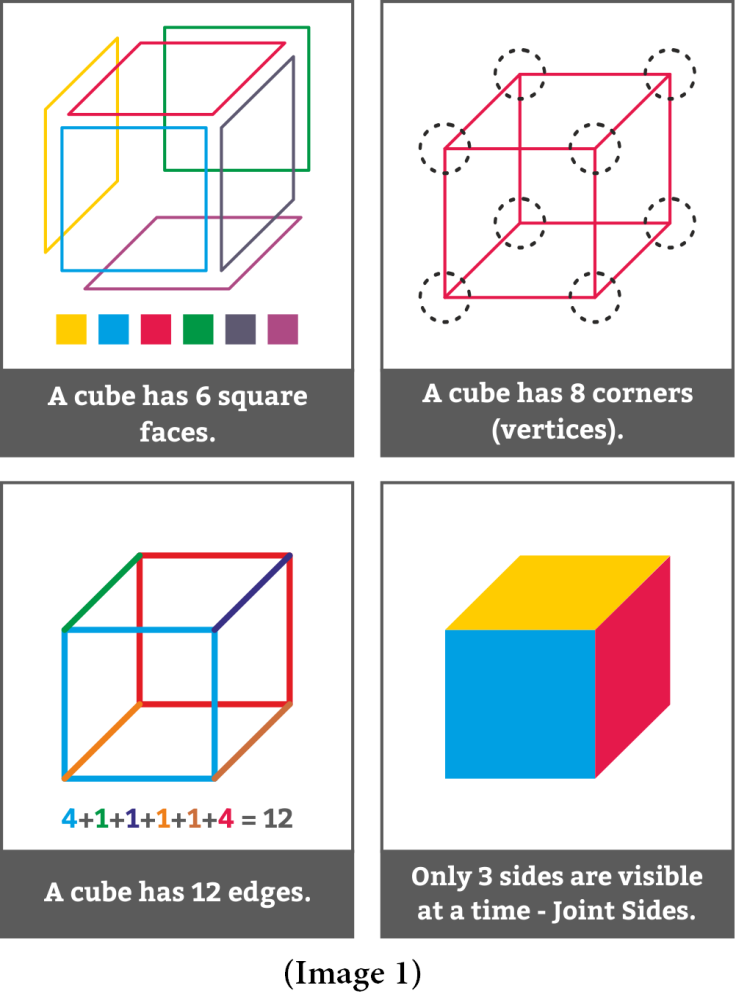• A cube has $$6$$ square faces or sides. As shown in the adjacent picture in yellow, blue, red, green, indigo and violet colour.
• A cube has $$8$$ points (vertices).
• A cube has $$12$$ edges. See the adjacent picture with $$4$$ blue edges, $$4$$ red edges, $$1$$ green, $$1$$ indigo, $$1$$ orange, $$1$$ brown. This makes a total of $$12$$.
• Only $$3$$ sides of a cube are visible at a time (known as “Joint Sides”) and these sides can never be on the opposite side of each other.
• Things that are shaped like a cube are often referred to as ‘cubic’.
• Most dice are cube shaped, with the numbers $$1$$ to $$6$$ on the different faces.

## Types of cubes:

1. Counting cubes.

2. Identifying different faces of a dice.

3. Identifying the faces of a net.

Counting Cubes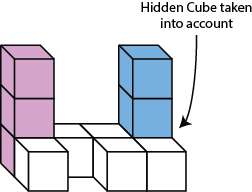Let’s count the cubes in the adjacent figure. Starting from the left top corner, what we can easily count is $$3$$ purple cubes + $$5$$ white cubes + $$2$$ blue cubes. This makes a total of $$10$$ cubes. But $$10$$ is the wrong answer. We have to consider the cube which is not seen too. That is the cube under the blue cube stack. So that gives us a total of $$10+1$$ (hidden cube) $$= 11.$$

There are a total $$11$$ cubes in the adjacent figure.

• Example:

Count the number of cubes in the given figure. (Assume there are hidden cubes in the given figures.)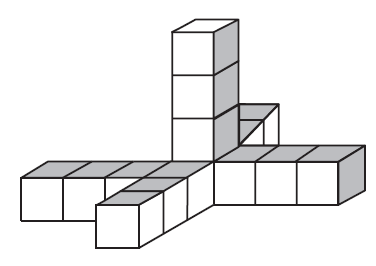(A) $$18$$             (B) $$15$$                 (C) $$12$$                 (D) $$14$$

 (B) $$15$$

Identifying different faces of a dice.

For example, if we need to know the number of dots opposite to $$1$$ dot.

Step 1:  Cancel out which are adjacent as they cannot be opposite if they are adjacent. So cancel $$5$$ dots, $$4$$ dots, $$3$$ dots looking at the figure.

Step 2 : Make a note of what is left. In our case $$2$$ dots and $$6$$ dots are left.

Step 3 : Let us assume it's $$2$$ dots which is opposite $$1$$ dot. So the adjacent sides of $$2$$ dots should be $$5$$ dots, $$4$$ dots, $$3$$ dots and $$6$$ dots. $$6$$ dots and $$4$$ dots are clearly adjacent as shown in the last position on the given figure. We are left with $$5$$ dots and $$3$$ dots.

Step 4 : $$3$$ dots is definitely adjacent to $$2$$ dots as it is not adjacent to $$4$$ dots as per the $$2$$ positions of $$4$$ dots seen in the figure. And if $$3$$ dots are adjacent to $$2$$ dots, then $$5$$ dots is definitely adjacent to $$2$$ dots because $$3$$ dots and $$5$$ dots are adjacent to each other as seen in the figure.

Step 5: Thus our assumption is proved and the answer is that it's $$2$$ dots opposite $$1$$ dot.

Note: Also the opposites are adjacent.

Example:

A die is thrown three times and its different positions are given below. How many dots lie opposite to two dots?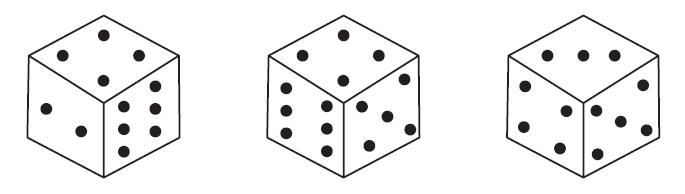(A) $$1$$             (B) $$3$$               (C) $$5$$         (D) $$6$$

 (C) $$5$$

Identifying the faces of a net.

Net --Two-dimensional shape that can be folded into a three-dimensional object is a net of that object. Left hand side is the net of the dice shown in the below image.

## Facts about cube and net

Solid $$3$$ dimensional cube when opened along its edges gives us a $$2$$ dimensional shape which is called a Net for that cube. Refer to the adjacent image.

There can be more than one way of unfolding the cube as shown in the adjacent image. (6 ways are illustrated here. There can be $$11$$ different nets for the same cube.)

The faces sharing a common edge will be adjacent faces of the cube. For example in the below image of the net of a dice, $$3$$ dots and $$2$$ dots, $$2$$ dots and $$1$$ dot, $$2$$ dots and $$4$$ dots, $$2$$ dots and $$6$$ dots share common edges. So the faces with $$3, 1, 4$$ and $$6$$ dots will be adjacent to faces with $$2$$ dots as shown in the image of the dice.

Opposite faces will not show on the same side of the cube. For example $$4$$ dots and $$3$$ dots for the below dice.

Example:

Which is the number opposite to $$4$$ when the given net of the cube is folded?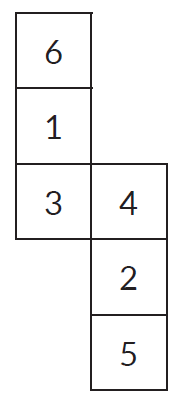(A) $$2$$                     (B) $$1$$                                      (C) $$5$$                     (D)  $$6$$

 (C) $$5$$

## Problems

### Question 1:

These questions are based on the given data.
A larger cube is painted on all six faces and then cut into a certain number of smaller but identical cubes. It was found that among the smaller cubes, there were $$8$$ cubes which had no face painted at all.

1.How many small cubes have exactly one face painted? (Hint: Exclude the corner ones)

(A) $$12$$             (B) $$24$$                     (C) $$16$$                           (D) $$32$$

### Question 2:

These questions are based on the given data.

A larger cube is painted on all six faces and then cut into a certain number of smaller but identical cubes. It was found that among the smaller cubes, there were $$8$$ cubes which had no face painted at all.

2. How many small cubes have exactly three faces painted? (Hint: Count the corners)

(A) $$0$$             (B) $$8$$            (C) $$27$$           (D) $$64$$

### Question 3:

Count the number of cubes in the given figure. (Assume there are hidden cubes in the given figures.)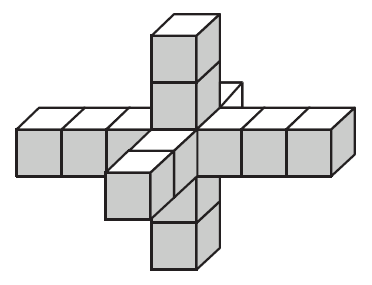(A) $$13$$             (B) $$11$$             (C) $$12$$             (D) $$14$$

### Question 4:

Three orientations of the same dice are shown. Which of the following symbols appears exactly opposite to symbol “=”?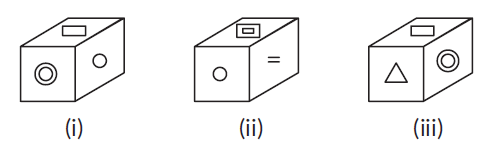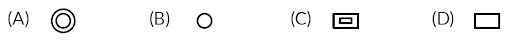### Question 5:

Choose the box that is like the box formed from the given sheet (X) of paper.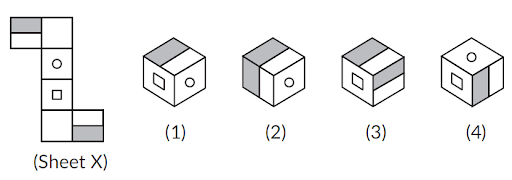(A) $$1$$ only      (B) $$1, 2$$ and $$4$$ only      (C) $$1$$ and $$4$$ only     (D) $$1, 2$$ and $$3$$ only

### Question 6:

The three different positions of a dice are given below:

Which number is on the face opposite to $$1$$?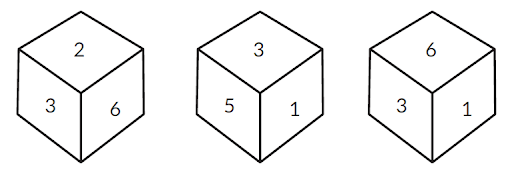(A) $$6$$          (B) $$2$$         (C) $$3$$         (D) $$5$$

### Question 7:

Choose a box that is similar to the box formed when the given sheet of paper is folded.